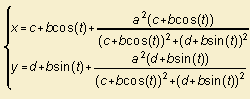# Joukowski curve

## trigonometric

last updated: 2003-08-01where z and z are in complex notation and z is on a circle, with given radius and center.

Let (c, d) be the center of the circle with radius b, then the curve can be written in parametric notation as 1):The curve is used for studying airfoil profiles. James and James (1992) state that then the point z=1 has to be inside the circle.

Some typical Joukowski forms are the following:

notes

1) let z = (x, y) = (c + b cos(t), d + b sin(t)), then work out the expression for z.